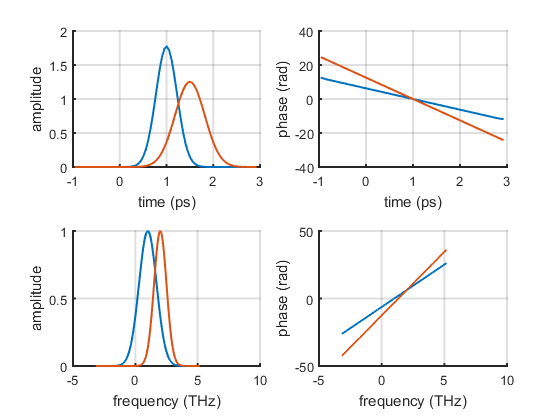# LaserPulse

LaserPulse is a Matlab class for streamlining simulations in nonlinear and ultrafast optics. It allows to store pulses in a convenient way, analyze them and perform simple mathematical operations.

## Pulses in time domain

A LaserPulse object can be initialized in either time of frequency domain. In either case both domains are computed and kept automatically synchronized.

The syntax for initializing a pulse in time domain is :

LaserPulse(time_array, time_units, complex_electric_field)

Where time_array is a Nx1 array of time steps, time_unit is a SI time unit (e.g. 'fs', 'ms', 's',...), and complex_electric_field is the time domain complex electric field expressed as a NXM matrix, where N is the number of time steps and M is the number of sub-pulses.

% time domain pulse:
t = (-10 : 0.01 : 9.99)';
p1 = LaserPulse(t, 'fs', exp(-t.^2 - 2i*pi*t));


In alternative, it is also possible to separately provide amplitude and phase of the electric field. This can be convenient to minimize phase wrapping.

LaserPulse(time_array, time_units, electric_field_amplitude, electric_field_phase)

## Pulses in frequency domain

The syntax for initializing a pulse in frequency domain is similar to the one for the time domain. Frequency units are in the form 'xHz', where 'x' is a SI prefix or the empty string (e.g. 'mHz', 'Hz', 'GHz')

% frequency domain pulse:
f = (-10 : 0.01 : 9.99)';
p2 = LaserPulse(f, 'PHz', exp(-(f-2).^2 + 2i*pi*f));


## Physical properties

The LaserPulse class provides several dependent properties for automatically calculating physical quantities like pulse duration, bandwidth and central frequency.

% display pulse duration for p1 and p2
fprintf('pulse durations are %.2f %s and %.2f %s\n', ...
p1.duration, p1.timeUnits, p2.duration, p2.timeUnits);

pulse durations are 1.18 fs and 0.38 fs

% display pulse bandwidth for p1 and p2
fprintf('pulse bandwidths are %.2f %s and %.2f %s\n', ...
p1.bandwidth, p1.frequencyUnits, p2.bandwidth, p2.frequencyUnits);

pulse bandwidths are 0.38 PHz and 1.18 PHz

% display central frequency for p1 and p2
fprintf('central frequencies are %.2f %s and %.2f %s\n', ...
p1.centralFrequency, p1.frequencyUnits, ...
p2.centralFrequency, p2.frequencyUnits);

central frequencies are 1.00 PHz and 2.00 PHz


## Names and definitions

The LaserPulse class uses extended names in order to clearly distinguish between fields in time and frequency domain. The fields are defined as follows:

p.temporalField == p.temporalAmplitude * exp(1i * p.temporalPhase)

p.spectralField == p.spectralAmplitude * exp(1i * p.spectralPhase)


Internally the fields are synchronized using a fft routine. The sign convention for the fft is: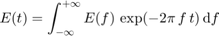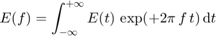Note that the sign convention is the one used in physics, i.e. the (-i) Fourier transform is used to pass from frequency domain to time domain, and the (+i) Fourier transform is used to pass from time domain to frequency domain.

## Plotting fields

In the LaserPulse class the plot() method is overloaded to provide a quick way to visualize fields in time and frequency domain. The plot() method returns a 4x1 array containing the axes handles of the four subplots.

h = p1.plot();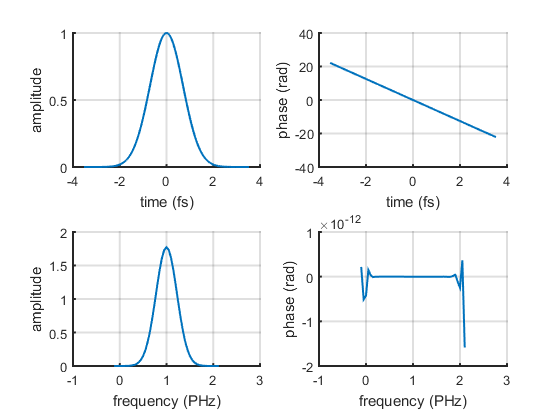A second pulse can be plotted on the top of the first one, by calling plot() with the axes handle array as second argument:

p2.plot(h);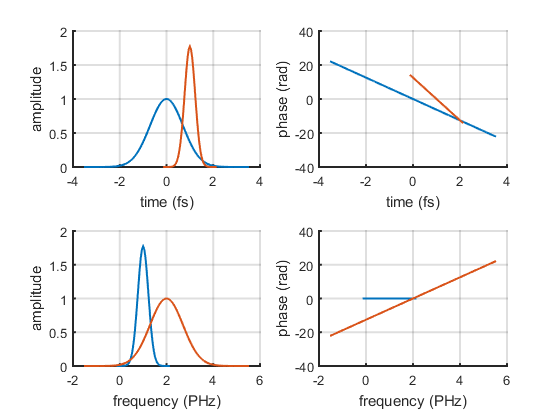More plot types can be obtained using the standard matlab commands. For instance, the next figure shows how to display the real part of the electric field in time domain:

figure()
plot(p1.timeArray, real(p1.temporalField), ...
p2.timeArray, real(p2.temporalField), ...
'LineWidth', 1.5);
xlim([-2, 2]*p1.duration)
xlabel(sprintf('time (%s)', p1.timeUnits));
ylabel('E(t)');
legend('p1', 'p2')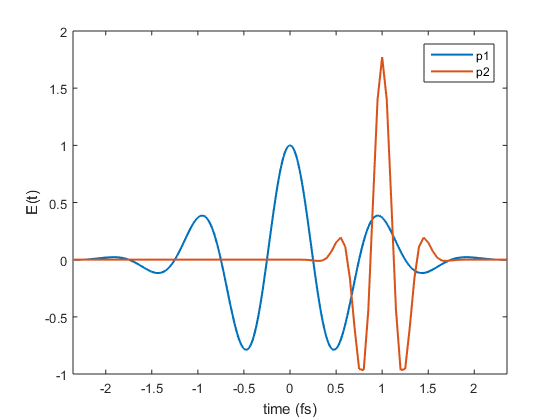## Sub-pulses

A LaserPulse object can contain multiple sub-pulses stacked in a multi-dimensional array. The first dimension is reserved for the the time/frequency axes.

f = (-10 : 0.01 : 9.99)';
% stacking two sub-pulses as array columns
p2 = LaserPulse(f, 'THz', ...
[exp(-(f-1).^2 + 2i*pi*f), exp(-2*(f-2).^2 + 3i*pi*f)]);
plot(p2)